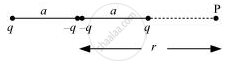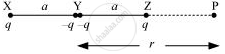Share

# For a Point on the Axis of the Quadrupole, Obtain the Dependence of Potential On R For R/A >> 1 - CBSE (Science) Class 12 - Physics

ConceptPotential Energy in an External Field Potential Energy of a Dipole in an External Field

#### Question

Figure 2.34 shows a charge array known as an electric quadrupole. For a point on the axis of the quadrupole, obtain the dependence of potential on for r/>> 1, and contrast your results with that due to an electric dipole, and an electric monopole (i.e., a single charge).#### Solution

Four charges of same magnitude are placed at points X, Y, Y, and Z respectively, as shown in the following figure.A point is located at P, which is r distance away from point Y.

The system of charges forms an electric quadrupole.

It can be considered that the system of the electric quadrupole has three charges.

Charge +placed at point X

Charge −2q placed at point Y

Charge +q placed at point Z

XY = YZ = a

YP = r

PX = r + a

PZ = r − a

Electrostatic potential caused by the system of three charges at point P is given by,

V=1/(4piin_0)[q/(XP)-(2q)/(YP)+q/(ZP)]

=1/(4piin_0)[q/(r+a)-(2q)/r+q/(r-a)]

=q/(4piin_0)[(r^2-ra-2r^2+2a^2+r^2+ra)/(r(r^2-a^2))]=q/(4piin_0)[(2a^2)/(r(r^2-a^2))]

=(2qa^2)/(4piin_0r^3(1-a^2/r^2))

Sincer/a>>1,

therefore a/r <<1

a^2/r^2is taken as negligible.

therefore V=(2qa^2)/(4piin_0r^3)

It can be inferred that potential, Vprop1/r^3

However, it is known that for a dipole, Vprop1/r^3

And, for a monopole, Vprop1/r^3

Is there an error in this question or solution?

#### APPEARS IN

NCERT Solution for Physics Textbook for Class 12 (2018 to Current)
Chapter 2: Electrostatic Potential and Capacitance
Q: 22 | Page no. 90
Solution For a Point on the Axis of the Quadrupole, Obtain the Dependence of Potential On R For R/A >> 1 Concept: Potential Energy in an External Field - Potential Energy of a Dipole in an External Field.
S